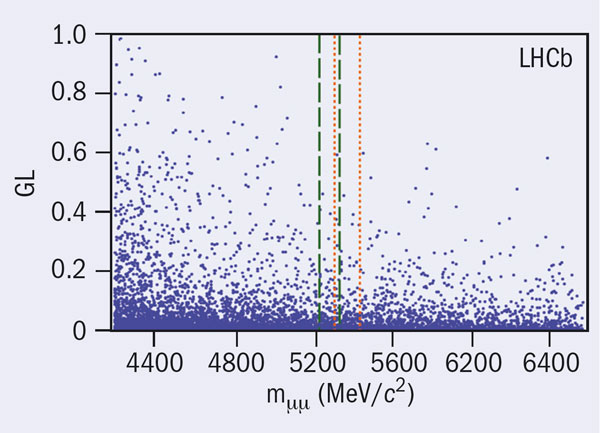# LHCb sets limits on rare B decays to dimuons

30 March 2011Scatter plot of the geometrical likelihood (GL) – a quantity that combines information from the vertex structure of the event and the transverse momentum of the B candidate – versus the invariant mass of selected muon pairs. The search windows for the signal regions for the B0 and B0s decays are marked with dashed and dotted lines respectively. Signal events would be uniformly distributed in GL, whereas background comes to a peak at low GL. The observed distribution is consistent with being only background.

The decay of B0 and B0s mesons exclusively to dimuons (μ+μ) is one of the most important channels in the search for new physics in the flavour sector at the LHC. In the Standard Model, the decays are rare because they can proceed only by processes involving loop diagrams and are in addition helicity-suppressed. However, new particles, in particular those that arise in models with an extended Higgs sector, can augment the decay rates and thus provide signs of new physics.

LHCb has published its first results for this important channel after searching for dimuon decays of both B0 andB0s in data collected at the LHC in proton–proton collisions at 7 TeV in the centre-of-mass. For the analysis, the collaboration used data from an integrated luminosity of around 37 pb–1 collected between July and October 2010.

They find no signal for either of the dimuon decays in this data sample: the observed numbers of events are consistent with the expectations for background. This allows the collaboration to place upper limits on the branching ratios for the two decays: B(B0s → μ+μ) < 5.6 × 10–8 and B(B0 → μ+μ) < 1.5 × 10–8 at 95% confidence level (CL). This is to be compared with Standard Model expectations of B(B0s → μ+μ) = 0.3 × 10–8 and B(B0 → μ+μ) = 0.01 × 10–8.

While there have previously been searches for B0(s) → μ+μ at e+e colliders, the highest sensitivity has so far been achieved at Fermilab’s Tevatron, thanks to the large bb– cross-section at hadron colliders. The most restrictive published limits at 95% CL come from the DØ collaboration’s analysis of 6.1 fb−1, yielding B(B0s → μ+μ) < 5.1 × 10–8, and the CDF collaboration’s analysis of 2 fb−1 yielding B(B0s → μ+μ) < 5.8 × 10–8 and B(B0 → μ+μ) < 1.8 × 10–8.

With less than 40 pb–1, LHCb has therefore already approached the sensitivity of existing measurements. This was possible thanks to the large acceptance and trigger efficiency of the experiment, as well as the increase in the bb cross-section at the higher energy of the LHC. With a much larger amount of data expected in 2011, the experiment should be able to explore smaller branching ratios, down to the interesting level of 10–8.